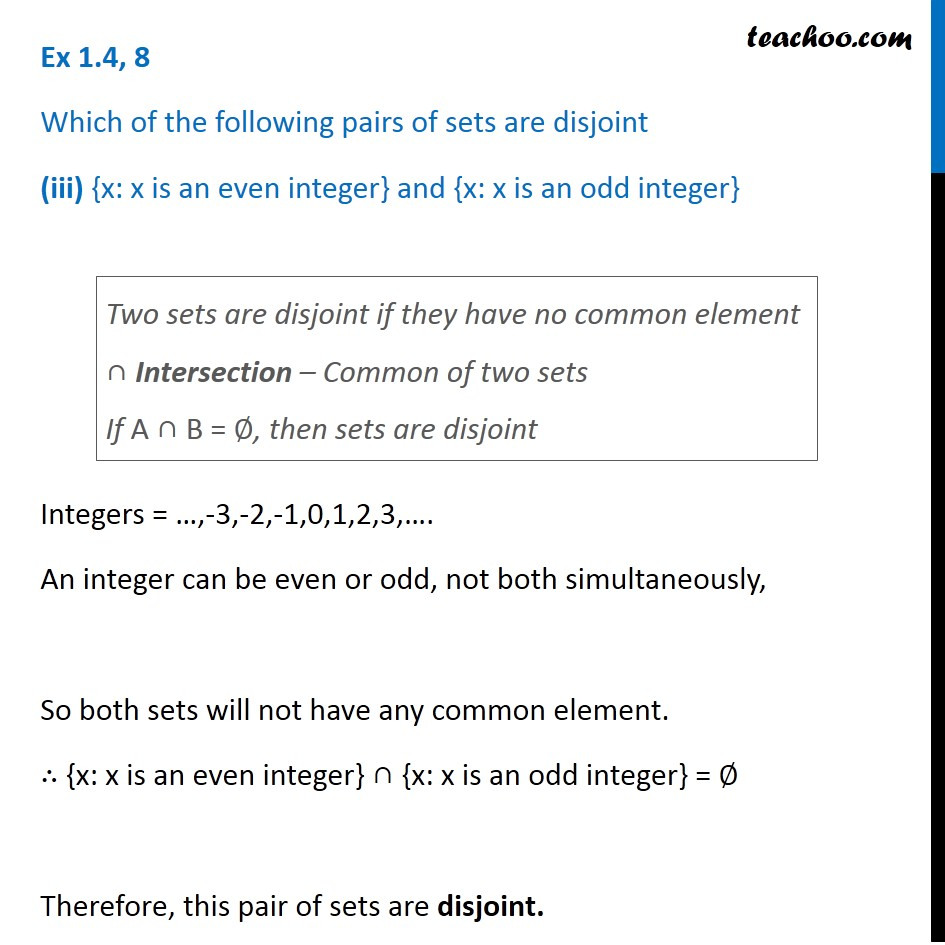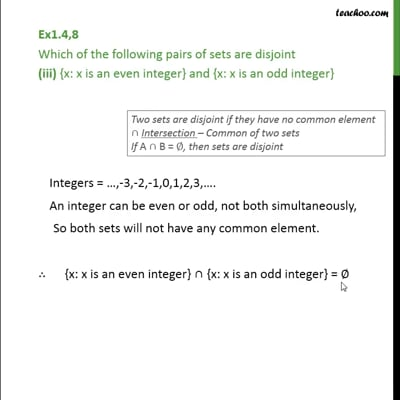Ex 1.4

Chapter 1 Class 11 Sets
Serial order wiseThis video is only available for Teachoo black users

Maths Crash Course - Live lectures + all videos + Real time Doubt solving!

### Transcript

Ex 1.4, 8 Which of the following pairs of sets are disjoint (iii) {x: x is an even integer} and {x: x is an odd integer} Integers = …,-3,-2,-1,0,1,2,3,…. An integer can be even or odd, not both simultaneously, So both sets will not have any common element. ∴ {x: x is an even integer} ∩ {x: x is an odd integer} = ∅ Therefore, this pair of sets are disjoint. Two sets are disjoint if they have no common element ∩ Intersection – Common of two sets If A ∩ B = ∅, then sets are disjoint